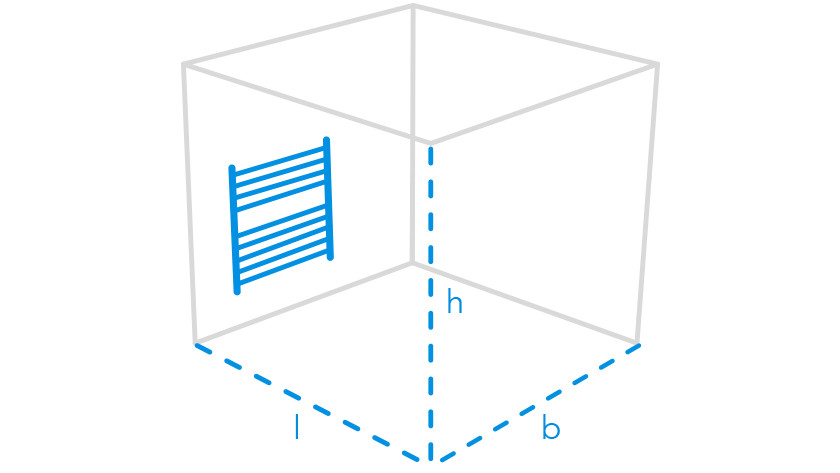Written by Leonie

Calculate here how much power a radiator needs to heat a room. The power in number of watts is an important indicator for the suitable radiator for you. If a radiator does not have enough power, it will not be warm enough in your room. If a radiator has more power than you need, then that is a waste of the money and of the extra space that the device occupies. The required power depends on 3 factors, namely the size of the room you want to heat in m3, the desired room temperature and the extent to which your house is insulated.

## You calculate the assets by:

Step 1. Do the length x width x height of your space and there is a number in m3. Step 2. Check the required number of watts per m3 for the space.

Step 3. Multiply the content in m3 with this number of watts.

Step 4. A correction factor? Add or subtract this from the total wattage.

Step 5. You now have the required power in watts for your new radiator.

content in m3 x required watts per m3 = x number of watts plus or minus correction factor in% = required power in watts.

## Step 1. The size of the room you want to heat in m3The larger the room you want to heat, the more power you need. That is not higher mathematics. You calculate the size of the room by taking a ruler and doing length x width x height in meters.

## Step 2. and 3. The desired room temperature

The recommended room temperature in a certain room is a general given. We agree that the rule of thumb below is correct for when you want it warm in the space concerned.

Space Recommended temperature Required number of watts per m3
Bathroom 24 degrees 93 watts
Living room 22 degrees 85 watts
Study 22 degrees 85 watts
Kitchen 20 degrees 77 watts
Bedroom 18 degrees 70 watts

## Step 4. To what extent your house is isolated and other correction factors

Every house is different. You have new houses that are well insulated or old houses filled with cracks and cracks in the insulation layer. This affects how much power you need to get it warm in a room. Corner and detached houses require more capacity. The same applies when you heat higher than the recommended temperature in a room, this often happens in retirement homes. Keep the correction factors in the table.

Correction factor Percentage correction
2 ° C higher temperature + 10%
Well insulated walls -10%
Poor wall insulation + 15%
Corner and detached house + 10%

## Example calculation

You have a bedroom of 20m3 where you want to mount a radiator. How much power do you need to get a pleasant temperature in your bedroom?

• Step 1. Do lx bxh of your space and there comes a number in m3. * \ NWe are talking about a space of 20m3.

• Step 2. Check the required number of watts per m3 for the room. *

• Step 3. Multiply the content in m3 by this number of watts. * A bedroom has a recommended temperature of 24 degrees and a required number of watts per m3 of 70 watts. Wired wattage = 20 x 70 watts = 1400 watts.

• Step 4. A correction factor? Add or subtract this from the total wattage. * You live in a new apartment with well insulated walls. Correction factor is therefore -10% of the total number of watts. 1400 watt - 10% = 1260 watt

• Step 5. You now have the power required in watt for your new radiator. Time to find a nice one. *Article by: